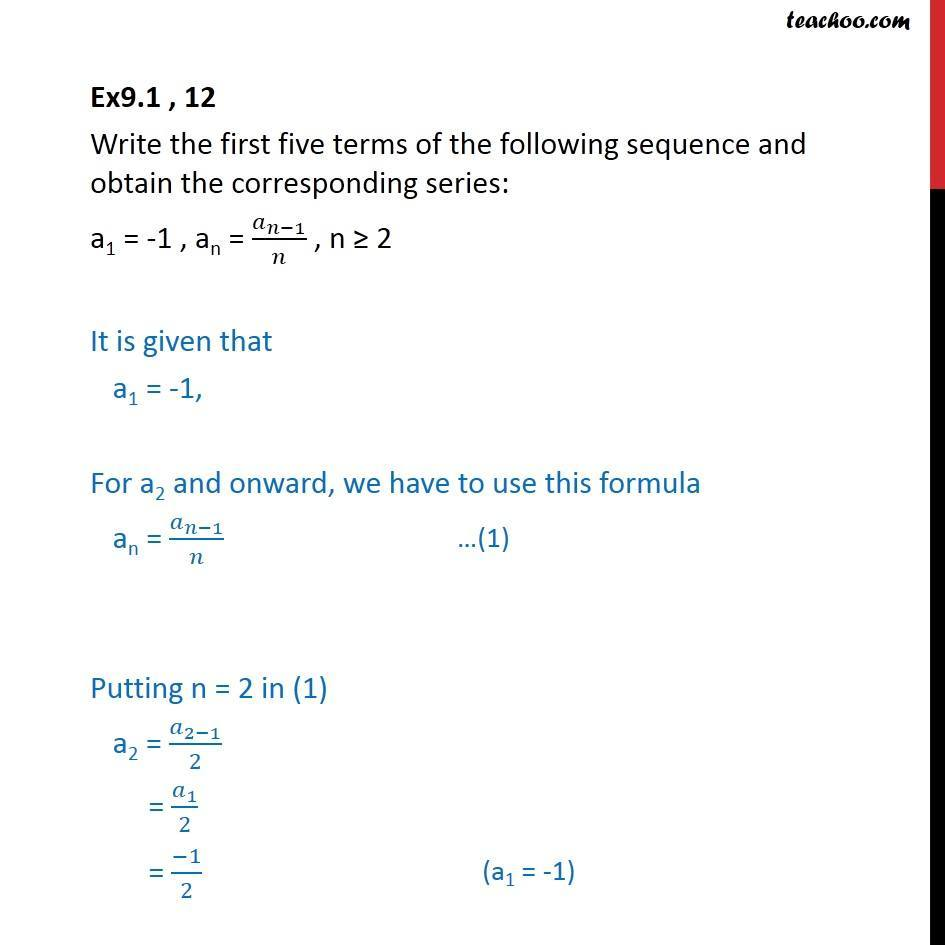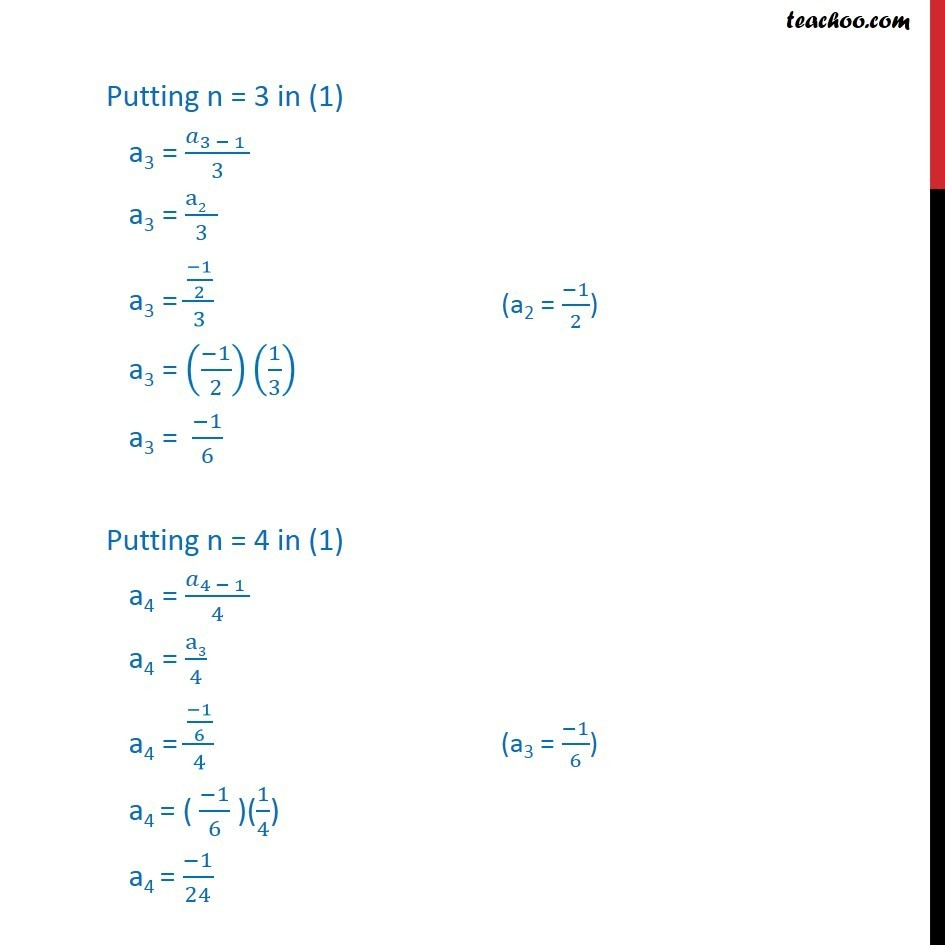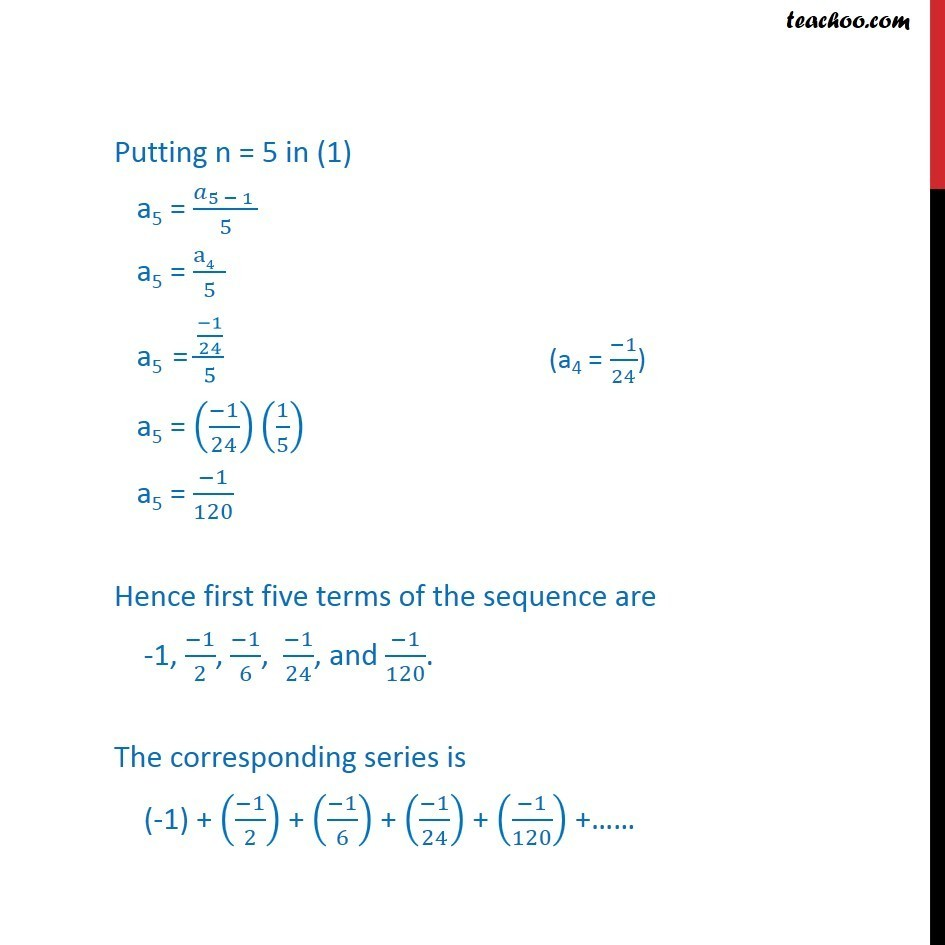Ex 8.1

Chapter 8 Class 11 Sequences and Series
Serial order wiseLearn in your speed, with individual attention - Teachoo Maths 1-on-1 Class

### Transcript

Ex9.1 , 12 Write the first five terms of the following sequence and obtain the corresponding series: a1 = -1 , an = 𝑎_(𝑛−1)/𝑛 , n ≥ 2 It is given that a1 = -1, For a2 and onward, we have to use this formula an = 𝑎_(𝑛−1)/𝑛 Putting n = 2 in (1) a2 = 𝑎_(2−1)/2 = 𝑎_1/2 = (−1)/2 Putting n = 3 in (1) a3 = 𝑎_(3 − 1 )/3 a3 = (a2−)/3 a3 = ((−1)/2)/3 a3 = ((−1)/2)(1/3) a3 = (−1)/6 Putting n = 4 in (1) a4 = 𝑎_(4 − 1 )/4 a4 = a3/4 a4 = ((−1)/6)/4 a4 = ( (−1)/6 )(1/4) a4 = (−1)/24 Putting n = 5 in (1) a5 = 𝑎_(5 − 1 )/5 a5 = (a4−)/5 a5 = ((−1)/24)/5 a5 = ((−1)/24)(1/5) a5 = (−1)/120 Hence first five terms of the sequence are -1, (−1)/2, (−1)/6, (−1)/24, and (−1)/120. The corresponding series is (-1) + ((−1)/2) + ((−1)/6) + ((−1)/24) + ((−1)/120) +……